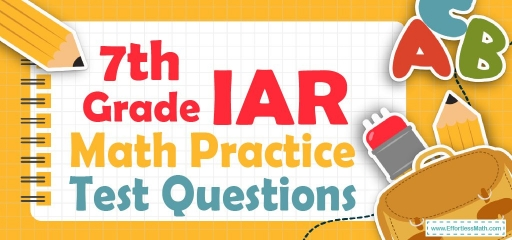# 7th Grade IAR Math Practice Test QuestionsHave you fully reviewed the math content of the 7th-grade IAR exam? Now it’s time to test your math skills!
The questions in this article are a collection of 10 of the most common questions in the 7th-grade Illinois Assessment of Readiness (IAR) math test.

These questions cover the most important math concepts of the 7th-grade IAR exam. Using these questions, 7th-grade students can assess themselves and realize how well they master the concepts of the 7th-grade IAR math test.

If you are a 7th-grade student and you want to take an important step in increasing your score, do not miss these 10 best questions!

Make sure to follow some of the related links at the bottom of this post to get a better idea of what kind of mathematics questions students need to practice.

The Absolute Best Book to Ace 7th Grade IAR Math Test

## 10 Sample 7th Grade IAR Math Practice Questions

1- A chemical solution contains $$4\%$$ alcohol. If there are $$24 ml$$ of alcohol, what is the volume of the solution?

A. $$240 ml$$

B. $$480 ml$$

C. $$600 ml$$

D. $$1200 ml$$

2- The price of a laptop is decreased by $$10\%$$ to $$360$$. What is its original price?

A. $$320$$

B. $$380$$

C. $$400$$

D. $$450$$

3- What is the median of these numbers? $$4, 9, 13, 8, 15, 18, 5$$

A. $$8$$

B. $$9$$

C. $$13$$

D. $$15$$

4- Three times the price of a laptop is equal to five times the price of a computer. If the price of the laptop is $$200$$ more than a computer, what is the price of the computer?

A. $$300$$

B. $$500$$

C. $$800$$

D. $$1500$$

5- What is the perimeter of a square that has an area of $$595.36$$ feet? ________

6- Jason is 9 miles ahead of Joe running at $$5.5$$ miles per hour and Joe is running at the speed of $$7$$ miles per hour. How long does it take Joe to catch Jason?

A. $$3$$ hours

B. $$4$$ hours

C. $$6$$ hours

D. $$8$$ hours

7- $$55$$ students took an exam and $$11$$ of them failed. What percent of the students passed the exam?

A. $$20 \%$$

B. $$40 \%$$

C. $$60 \%$$

D. $$80 \%$$

8- Jason needs an $$75\%$$ average in his writing class to pass. On his first $$4$$ exams, he earned scores of $$68\%, 72\%, 85\%$$, and $$90\%$$. What is the minimum score Jason can earn on his fifth and final test to pass? __________

9- A bank is offering $$3.5\%$$ simple interest on a savings account. If you deposit $$12,000$$, how much interest will you earn in two years?

A. $$420$$

B. $$840$$

C. $$4200$$

D. $$8400$$

10- $$5$$ less than twice a positive integer is $$83$$. What is the integer?

A. $$39$$

B. $$41$$

C. $$42$$

D. $$44$$

Best 7th Grade IAR Math Prep Resource

1- C
$$4\%$$ of the volume of the solution is alcohol. Let $$x$$ be the volume of the solution.
Then: $$4\% \space of \space x = 24 \space ml ⇒ 0.04 x = 24 ⇒ x = 24 ÷ 0.04 = 600$$

2- C
Let $$x$$ be the original price.
If the price of a laptop is decreased by $$10\%$$ to $$360$$, then:
$$90 \% of x=360 ⇒ 0.90x=360 ⇒ x=360÷0.90=400$$

3- B
Write the numbers in order:
$$4, 5, 8, 9, 13, 15, 18$$
Since we have $$7$$ numbers ($$7$$ is odd), then the median is the number in the middle, which is $$9$$.

4- A
Let $$L$$ be the price of a laptop and $$C$$ be the price of a computer.
$$3(L) =5(C) \space and \space L = 200 + C$$
Therefore, $$3(200 + C) =5C ⇒ 600 + 3C = 5C ⇒ C=300$$

5- 97.6
Use the area of the square formula.
$$S = a^2 ⇒ 595.36 = a^2 ⇒ a = 24.4$$
Use the perimeter of the square formula.
$$P = 4a ⇒ P=4(24.4) ⇒ P = 97.6$$

6- C
The distance between Jason and Joe is $$9$$ miles. Jason running at $$5.5$$ miles per hour and Joe is running at the speed of $$7$$ miles per hour. Therefore, every hour the distance is $$1.5$$ miles less.
$$9 ÷ 1.5 = 6$$

7- D
The failing rate is $$11$$ out of $$55 = \frac{11}{55}$$.
Change the fraction to percent:
$$\frac{11}{55} ×100\%=20\%$$
$$20$$ percent of students failed. Therefore, $$80$$ percent of students passed the exam.

8- 60
Jason needs an $$75\%$$ average to pass five exams. Therefore, the sum of $$5$$ exams must be at least $$5 × 75 = 375$$
The sum of $$4$$ exams is:
$$68 + 72 + 85 + 90 = 315$$
The minimum score Jason can earn on his fifth and final test to pass is:
$$375 – 315 = 60$$

9- B
Use simple interest formula:
$$I=prt$$
(I = interest, p = principal, r = rate, t = time)
$$I=(12000)(0.035)(2)=840$$

10- D
Let $$x$$ be the integer. Then:
$$2x – 5 = 83$$
Add $$5$$ both sides: $$2x = 88$$
Divide both sides by $$2$$: $$x = 44$$

Looking for the best resource to help you succeed on the IAR Math Grade 7 Math test?

The Best Books to Ace 7th Grade IAR Math Test

### What people say about "7th Grade IAR Math Practice Test Questions - Effortless Math: We Help Students Learn to LOVE Mathematics"?

No one replied yet.

X
30% OFF

Limited time only!

Save Over 30%

SAVE $5 It was$16.99 now it is \$11.99